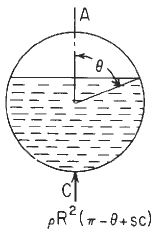Related Resources: calculators

### Circular Ring Moment Analysis #17 Roarks Formulas for Stress and Strain Equations and Calculator

Circular Ring Moment, Hoop Load, and Radial Shear Equations and Calculator #17.

Per. Roarks Formulas for Stress and Strain Formulas for Circular Rings Section 9, Reference, loading, and load terms #17. Formulas for moments, loads, and deformations and some selected numerical values.

Unit axial segment of pipe partly filled with liquid of weight per unit volume ρ and supported at the base.Circular Ring Loading #17Circular Ring Dimensional PropertiesResultant moment, hoop load, and radial shear

θ ≥ π / 2

Moment
M = MA - NA R ( 1 - u) + VA R z + LTM

Hoop Stress
N = NA u + Va z + LTN

V = - NA z + VA u + LTv

LTM LTN, and LTV are load terms for several types of load.Unit step function defined by use of ⟨ ⟩, See explanation at bottom of page.
⟨ x - θ ⟩0VA = 0

Ri / t ≥ 10 means thin wall for pressure vessels (per. ASME Pressure Vessel Code)

Hoop Stress Deformation Factor α

α = e / R for thick rings
α = I / AR2 for thin rings
A = π [ R2 - Ri2 ]

Transverse (radial) shear deformation factor β

β = 2F (1 + v) e / R for thick rings
β = FEI / GAR2 for thin rings

k1 = 1 - α + β

k2 = 1 - α

Plastic section modulus
F = Z / I c
Z = Zx = Zy = 1.33R3
Ix = Iy = ( π / 4 ) ( R4 - Ri4)

Deformation in the Horizontal Axis (Click for larger view)Deformation in the Vertical Axis (Click for larger view)Change of length of Circular Ring (Click for larger view)Where (when used in equations and this calculator):

v and w = unit loads (force per unit of circumferential length);
G = Shear modulus of elasticity
F = Shape factor for the cross section (= Z / I c)
Z = Plastic section modulus
w and v = unit loads (force per unit of circumferential length);
ρ = unit weight of contained liquid (force per unit volume);
Mo = applied couple (force-length);
MA, MB, Mc, and M are internal moments at A;B;C, and x, respectively, positive as shown.
NA N, VA, and V are internal forces, positive as shown.
E = modulus of elasticity (force per unit area);
v = Poisson’s ratio;
A = cross-sectional area (length squared);
R = Outside radius to the centroid of the cross section (length);
Ri = Inside radius to the centroid of the cross section;
t = wall thickness
I = area moment of inertia of ring cross section about the principal axis perpendicular to the plane of the ring (length4). [Note that for a pipe or cylinder, a representative segment of unit axial length may be used by replacing EI by Et3 / 12 (1 - v2 )
e ≈ I / ( R A) positive distance measured radially inward from the centroidal axis of the cross section to the neutral axis of pure bending
θ, x, and Φ are angles (radians) and are limited to the range zero to π for all cases except 18 and 19
s = sin θ
c = cos θ
z = sin x,
u = cos x
n = sin Φ
m = cos Φ
α - Hoop stress deformation factor
ΔDV = Change in vertical diameter (in, mm),
ΔDH = Change in horizontal diameter (in, mm),
ΔL = Change in lower half of vertical diameterthe vertical motion realative to point C of a line connecting points B and D on the ring,
ΔLW = Vertical motion relative to point C of a horizontal line connecting the load points on the ring,
ΔLWH = Change in length of a horizontal line connecting the load points on the ring,
ψ = angular rotation (radians) of the load point in the plane of the ring and is positive in the direction of positive θ.

Unit step function defined by use of ⟨ ⟩
⟨ x - θ ⟩0

if x < 0, ⟨ x - θ ⟩0 =0;
if x > 0, ⟨ x - θ ⟩0 =1;

At x = θ the unit step function is undefined just as vertical shear is undefined directly beneath a concentrated load. The use of the angle brackets ⟨ ⟩ is extended to other cases involving powers of the unit step function and the ordinary function ⟨ x - a ⟩n

Thus, the quantity ⟨ x - a ⟩n ⟨ x - a ⟩0 is shortened to ⟨ x - a ⟩n and again is given a value of zero if x < a and is ( x-a )n if x > a.

Supplemental formulas (not included in calculator)K Table of Constants (Click for larger view)Reference:

Roarks Formulas for Stress and Strain, 7th Edition, Table 9.2 Reference No. 17, loading, and load terms.